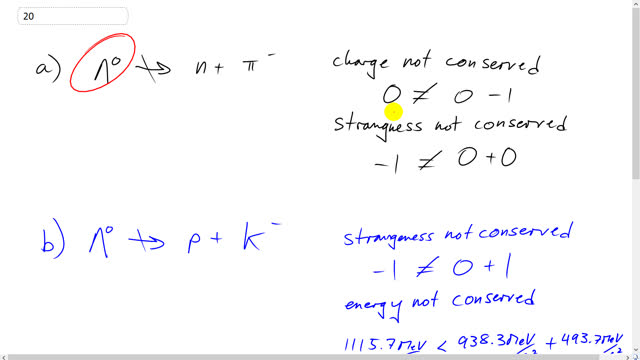## You are here

Question:

The $\Lambda^0$ cannot decay by the following reactions. What conservation laws are violated in each of the reactions?

1. $\Lambda^0 \nrightarrow$ n + $\pi^-$
2. $\Lambda^0 \nrightarrow$ p + $\textrm{K}^-$
3. $\Lambda^0 \nrightarrow \pi^+ + \pi^-$

Giancoli, Douglas C., Physics: Principles with Applications, 7th Ed., ©2014. Reprinted by permission of Pearson Education Inc., New York.
The question will be visible after logging in, as required by Pearson Education Inc.